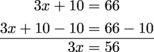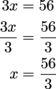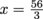## Equations and Word Problems

Audrey II is a man-eating plant living in the Little Shop of Horrors and it needs blood, and lots of it. Luckily its caretaker, Seymour, has discovered a blood substitute to feed Audrey II. Now that death is out of the way, he can figure out how fast Audrey II grows.

Audrey II is 10 inches tall and it grows a steady 3 inches a day for x days. How tall will it be in 7 days? How tall in 14 days?

We can write an expression for the growth rate of Audrey II by adding its current height (10 in.) to the product of how much it grows each day (3 in.) and the number of days it grows (x).

10 + 3x

To be consistent with traditional algebraic order, we'll write the variable term (3x) before the constant term (10).

3x + 10

Now that we have our expression, we can use it to see how tall Audrey II will be in 7 days and how tall it will be in 14 days.

When x = 7 we have:

3(7) + 10 =
21 + 10 =
31

So in 7 days, Audrey II will be 31 inches tall.

Now try it with x = 14.

3(14) + 10 =
42 + 10 =
52

In 14 days, Audrey II will be 52 inches tall. Yikes!

This expression can be used in an equation too. Seymour is 5 feet 6 inches tall, and he wants to know how many days it'll be before Audrey II is as tall as he is. We can set this expression equal to Seymour's height (5 feet + 6 inches) and then solve for x.

3x + 10 = 5 feet + 6 inches

First we need to get all our numbers in inches. Since Seymour is 5 feet 6 inches, he's 60 inches + 6 inches = 66 inches tall. Now, we solve the equation.

3x + 10 = 66

Subtract 10 from both sides.Then divide both sides by 3.Boom, we found out that, or about 18.7 days. So in about 18 and a half days, Audrey II will be taller than Seymour. Run Seymour, run!